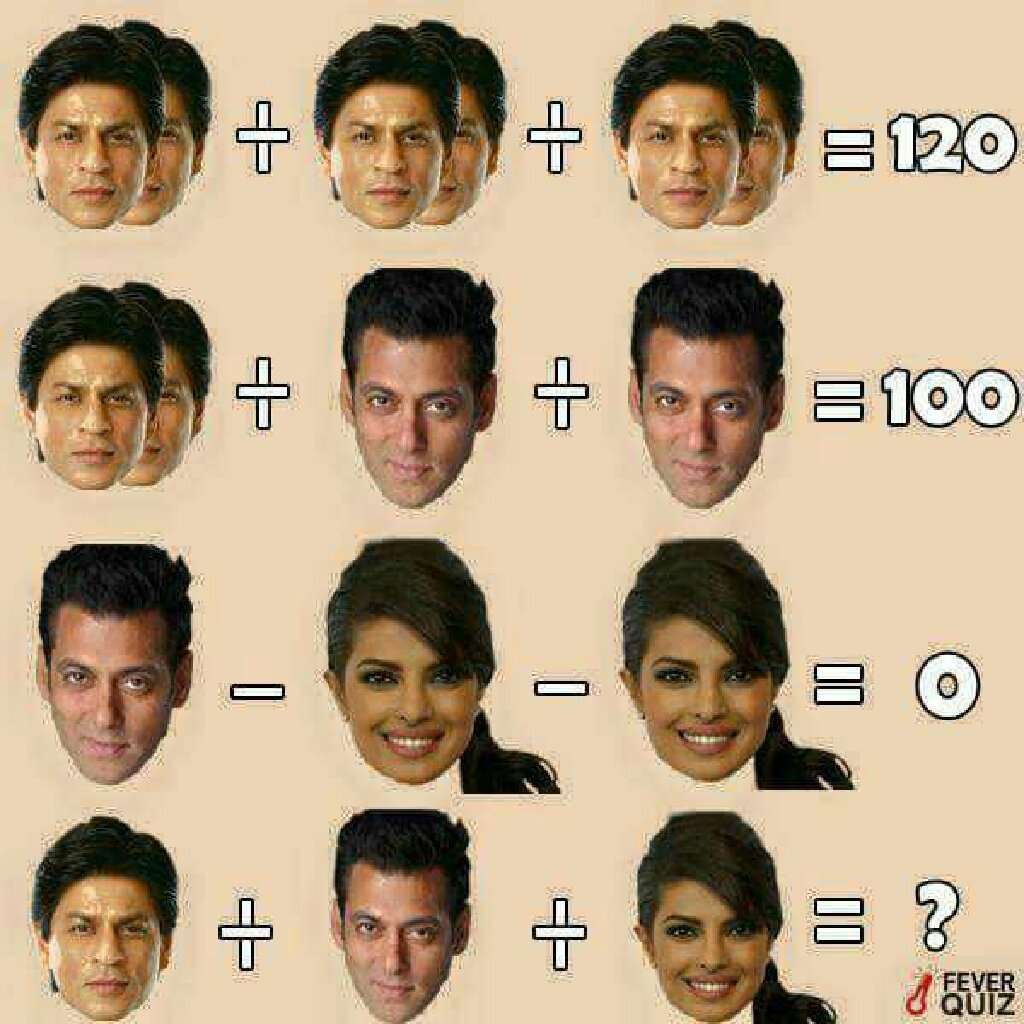jayp
over 3 years

if u but value of sharukh 40 then the equation for first will be
40×2+40×2+40×2=120
80+80+80=120
240=120 which is not correct.
@kiranmayikiranmayi
over 3 years

40+30+15=85jayp
over 3 years

20+30+15=65